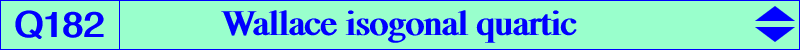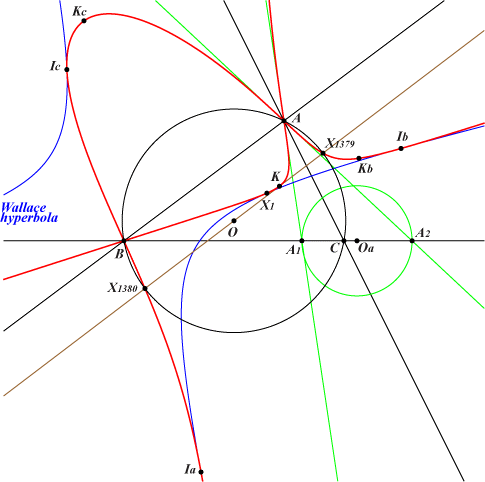∑ a^4 (b^2 – c^2) y^2 z^2 = 0X(1), X(6), X(19), X(64), X(1379), X(1380), X(1676), X(1677), X(2129), X(2576), X(2577), X(3224), X(3440), X(3441), X(3489), X(3490), X(8946), X(8948), X(14370), X(31956), X(34130), X(39797), X(39964), X(43739), X(52678) vertices Ia, Ib, Ic and Ka, Kb, Kc of the excentral and extangents triangles more generally, vertices of the anticevian triangle of any of its pointsQ182 is a quartic very similar to Q066 where a generalization is given. See the related Q124 whose barycentric product by X(6) is Q182. Q182 is the isogonal transform of the Wallace diagonal rectangular hyperbola which is the anticomplement of the Kiepert hyperbola. It contains X(1), X(2), X(20), X(63), X(147), X(194), X(487), X(488), X(616), X(617), X(627), X(628), X(1670), X(1671), X(1764), X(2128), X(2582), X(2583), X(2896), etc. Q182 is also the square root of the circum-conic with perspector X(669) passing through X(6), X(32), X(83), X(213), X(729), X(981), X(1918), X(1974), X(2207), X(2281), X(2422), X(3114), X(3224), X(3225), etc. In other words, Q182 contains the square roots of all the points on the conic. The points A, B, C are nodes. The nodal tangents pass through the traces of the circles orthogonal to the circum-circle and centered at the corresponding vertices of the cevian triangle OaObOc of X(669).